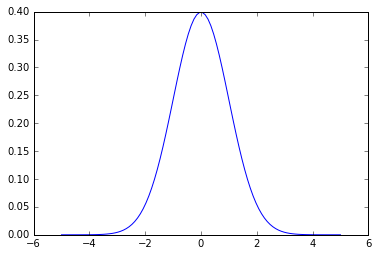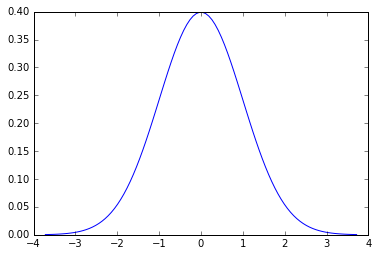Prev: Distributions       |      Next: The t distribution

The normal distribution¶

• Normal (a.k.a.: 'Gausian', 'bell curve') distribution is of major importance in statistics and in hypothesis testing specifically. The probability density function for normal distribution in scipy is written as:

norm.pdf(x) = exp(-x**2/2)/sqrt(2*pi)

Working with normal distribution¶

• What we present below as code work with the normal distributions is valid for other distributions too.

Import and get help¶

• To work with statistical distributions we import the scipy.stats package. Usually we import the whole scipy.stats package
• However, importing specific distribution classes is also possible
In :
import scipy.stats as stats   # Imports the entire scipy.stats (all distributions)

from scipy.stats import norm  # imports only the normal distribution

from scipy.stats import t     # imports only the Studnet's t distribution

from scipy.stats import f     # imports only the Fisher's f distribution
In :
# Get help by printing the distribution docstring as follows:
# print(stats.norm.__doc__)      # or print(stats.t.__doc__) etc.
# print(stats.norm.__doc__)

Simple statistical functions¶

• Import norm and use some basic distribution statistical functions like:

• median => median()
• mean => mean()
• standard deviation => std()
• variance => var()
• stats() returns the first four moments of the distribution: mean, std, skew and kyrtosis (for a reminder of what 'moments' are in statistics read here)

In :
from scipy.stats import norm

# get median, mean, standard deviation and var
print(norm.median(), norm.mean(), norm.std(), norm.var())

# or use stats() function
m, v, s, k = norm.stats(loc=0, scale=1, moments='mvsk')
print(m, v, s, k)
0.0 0.0 1.0 1.0
0.0 1.0 0.0 0.0
• But why do we get these '0's and '1's?

Standardized distribution - The loc' and 'scale' shape parameters¶

• If you do not do anything else distributions are in a standardized form using the standard scores (a.k.a: z scores, z values). A z score is calculated by the transformation formula:
• z = (x - M)/SD, where M: mean and SD: standard deviation

Thus, z scores are independent from any specific measurements and only describe how far from M is the x value in terms of SD

• Standardized also means that the mean and the standard deviation (SD) of the distribution are set to '0' and '1' respectively.
• If you want to shift and/or scale the distribution you have to pass arguments and give new values to the distribution shape parameters: loc and scale
• loc represents the mean
• scale represents SD
In :
# loc and scale examples

print(norm.var(loc=2, scale=4))    # prints the variance of a norm distro with M=2 and STD=4

# print the mean and variance of a norm distro with M=1.5 and STD=0.5
m, v = norm.stats(loc=1.5, scale=0.3, moments='mv')
print(m, v)
16.0
1.5 0.09

'Frozen' distribution¶

• When using the norm() constructor essentially we refer to a whole family of normal distributions with varied 'shape parameters' (mean and standard deviation)
• It is better to 'freeze' the distribution by constructing a non-varied normal distribution with specific M and SD.
• To do so, we need to call the norm() constructor and pass as arguments the values of loc and scale (that is, define M and SD)
• After this we can use the identifier (name) of the frozen distribution without cintinuously passing as arguments the shape parameters
In :
rv = norm(loc=2, scale=4)   # Use 'rv' to refer to the frozen norm distro with M=2 and SD=4
m, v, s, k = rv.stats(moments='mvsk')
print( m, v, s, k)
2.0 16.0 0.0 0.0

'rvs' : get random values from a distro¶

• We can get one or more random values from a distribution with the rvs (random variates) method or the scipy.randn() function.
In :
from scipy.stats import norm
obs = norm.rvs(loc=0, scale=1, size=10)
obs
Out:
array([-0.20632554,  0.14462284, -1.72352661,  0.92794621, -0.13120689,
0.0994114 , -0.54655402, -0.12889049, -0.98265513, -1.37893909])
• Similarly we can call the scipy.randn() function to get a number of random points form the normal distribution
• The describe() function can give us some sample statistics
In :
import scipy as sp
s = sp.randn(100)
sp.stats.describe(s)
Out:
DescribeResult(nobs=100, minmax=(-2.6409918708529556, 2.8694264964028888), mean=0.026156031493620554, variance=1.0577734502240392, skewness=0.01648751858664814, kurtosis=-0.40386278813255805)

How to plot a distribution using pdf()¶

In :
import numpy as np
from scipy.stats import norm
import matplotlib.pyplot as plt
%matplotlib inline

# A simplistic way to do it
x = np.linspace(-5,5,100)     # define a big enough x interval
nd = norm.pdf(x)              # get the norm.pdf for x interval
plt.plot(x,nd)                # plot it!
Out:
[<matplotlib.lines.Line2D at 0x81dff98>]• Note that: in a standardized form the area below the pdf curve is always equal to 1 (= the certainty that a x value has been measured). Changing M and SD results to changes in the distribution representation so that the area remains the same.
• Why is it simplistic? For one reason because we made the assumption that the [-5,5] interval fits well to describe the normal distribution (and we were right!).
• But what if we don't really know the practical limits of the x values for a specific distribution?
• The answer is that we always know that:
• ...for any distribution the ppf() function returns a x value that corresponds to the probability that this value appears.
• ...near the lower or higher limit of the value range of the distribution the cumulative probability (cdf) becomes very small (almost zero) or very large (almost 1) respectively.

Thus, the [ppf(0.0001), ppf(0.9999)] value range should return x values close enough to the lower or higher end of the x values of the distribution. So, we rewrite the code as follows:

In :
# A better approach

rv = norm(loc=0, scale=1)           # Freeze the norm distribution
x = np.linspace(rv.ppf(0.0001), rv.ppf(0.9999), 100)
y = rv.pdf(x)
plt.plot(x,y)

Out:
[<matplotlib.lines.Line2D at 0x860b198>]Probability less/greater than a cutoff value¶

• As already discussed, a common issue when using any distribution is testing whether the probability that the variate x takes a value that is less/greater than a cutoff probability 'a'
• Knowing this probability important conclusions can be drawn regarding hypothesis testing.
• Obviously, the sf function will help finding the answer.
In :
a = 0.05  # set the cutoff

rv = norm(loc=0, scale=1)
x = np.random.normal(size=1)

p = rv.sf(x) # equal but sometimes more accurate than '1-rv.cdf(x)'

if p < a:
print('Cutoff at: ', x, p)
else:
print('No cutoff', x, p)
No cutoff [-0.66950879] [ 0.74841451]